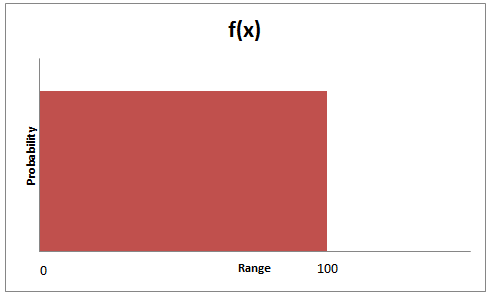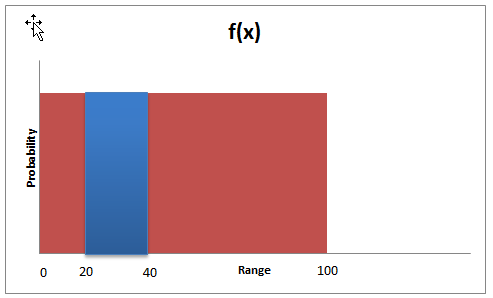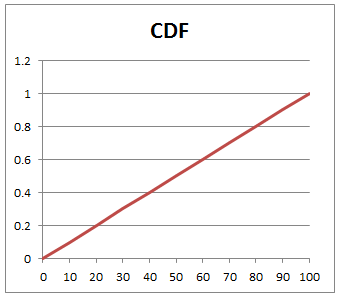# Continuous Uniform Distribution

We know that a discrete uniform random variable is a discrete random variable for which the probability of each outcome is the same. We also know that a random variable is continuous if it can take an infinite number of values between the possible values for the random variable.

Keeping these two characteristics in mind, we can say that a continuous random variable will have a uniform distribution if all the possible values in the given range have a uniform or same probability.

A continuous uniform distribution has the following characteristics.

• It has an infinite number of possible values between two possible values a and b.
• The probability for each outcome, P(X=x) or p(x) = 0
• The probability of an outcome outside the range is 0.
• The probability of an outcome being between two values n1 and n2 is simply the ratio of two ranges.

P(n1 <= X <= n2) = (x2-x1)/(b-a)

Example

If X is uniformly distributed between 0 and 100, it can be represented as follows:Let’s say you want to calculate the probability of the value falling between 20 and 40. This will be calculated as:

P(20 <=X <= 40) = (40 – 20)/(100 – 0) = 0.20

This is shown below:Since each possibility has an equal probability of occurring, the cumulative uniform distribution function will be a straight line, as shown below:Get our Data Science for Finance Bundle for just $29$51.